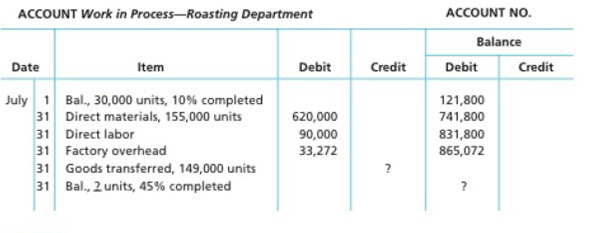Chapter 20, Problem 20.2APR

Chapter
Section
Textbook Problem

Cost of production reportArabica Highland Coffee Company roasts and packs coffee beans. The process begins by placing coffee beans into the Roasting Department. From the Roasting Department, coffee beans are then transferred to the Packing Department. The following is a partial work in process account of the Roasting Department at July 31:Instructions1. Prepare a cost of production report and identify the missing amounts for Work m Process—Roasting Department.2. Assuming that the July 1 work in process inventory includes $119.400 of direct materials, determine the increase or decrease in the cost per equivalent unit for direct materials and conversion between June and July. (a) To determine Process costs It is a method of cost accounting, which is used where the production is continuous, and the product needs various processes to complete. This method is used to ascertain the cost of the product at each process or stage of production. Equivalents units for production The activity of a processing department in terms of fully completed units is known as equivalent units. It includes the completed units of direct materials and conversion cost of beginning work in process, units completed and transferred out, and ending work in process. Production cost report A production cost report is a comprehensive report prepared for each department separately at the end of a particular period, which represents the physical flow and cost flow of product for the concerned department. To Determine: The equivalents units for production of direct materials and conversion costs for the month of January for Company AHC. Explanation Calculate the equivalents units for production of direct materials and conversion costs for the month of January for Company AHC as shown below: Figure (1) Working notes: Calculate opening work in process inventory for conversion costs as shown below: Opening work in process inventory for conversion costs) = 110%=90% Calculate units started and completed in July as shown below: Units started and completed in July = (Units received from weaving departmentEnding work in process inventory)=155,000units 36,000units=119,000units Calculate whole ending work in process inventory in July as shown below: Whole ending work in process inventory in July)=(Opening work in process inventory +Received from material storeroom Transferred to packing department)=30,000units +155,000units 149,000units=36,000units Calculate ending work in process inventory for conversion costs as shown below: Ending work in process inventory for conversion costs) = (Total units for ending work in proces inventory ×Completed percent for conversion costs)=36,000units ×45%=16,200units Equivalent units for production is calculated by adding units of opening work in process inventory, transferred to finished goods in august, and units for ending work in process inventory. Therefore, an equivalents unit for production for direct materials is 155,000 units and equivalent units for production for conversion costs is 162,200 units. Calculate direct materials and conversion cost equivalent cost per unit for July as shown below: Direct material cost per unit =Total direct material costsEquivalent units for direct materials=$620,000155,000units=$4.00Per unit Conversion cost per unit =Total conversion costsEquivalent units for conversion=$123,272162,200units=$0 (b) To determine Increase or decrease in the cost per equivalent per unit for direct materials and conversion cost between June and July when, work in process inventory as on July 1 includes$119,400 of direct materials.

Still sussing out bartleby?

Check out a sample textbook solution.

See a sample solution

The Solution to Your Study Problems

Bartleby provides explanations to thousands of textbook problems written by our experts, many with advanced degrees!

Get Started

What is a computer virus?

Accounting Information Systems

Why do economists make assumptions?

Brief Principles of Macroeconomics (MindTap Course List)

What is a loan amortization schedule, and what are some ways these schedules are used?

Fundamentals Of Financial Management, Concise Edition (mindtap Course List)

Define the term marketing

MKTG 12:STUDENT ED.-TEXT

DPS CALCULATION Warr Corporation just paid a dividend of 1.50 a share (that is, D0 = 150). The dividend is expe...

Fundamentals of Financial Management, Concise Edition (with Thomson ONE - Business School Edition, 1 term (6 months) Printed Access Card) (MindTap Course List)